## QED

### Gauge Invariance

1. Gauge Invariance in Classical Electrodynamics
Maxwell's equation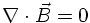suggests that there is a vector potential fulfilling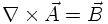The magnetic field is unchanged if one adds a gradient of an arbitrary scalar field Λ: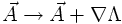Similar in line, the Maxwell equation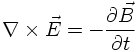suggests that there is a scalar potential V fulfilling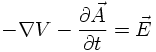In this case one can add a time derivative of an arbitrary scalar field Λ to the scalar potential Vwithout changing the electric field.

To summarize this in a covariant notation: The field-strength tensorwith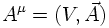is unchanged under a 'gauge transformation'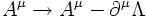with Λ(x) being an arbitrary function.
The same electrodynamics can be described by many different four-vector potentials. This is what is meant by GAUGE INVARIANCE of classical electrodynamics.

The two Maxwell equations from above are then rewritten as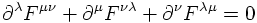The two remaining Maxwell equations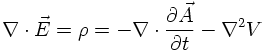and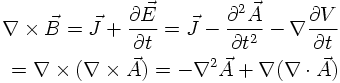can be written in the compact form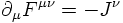with the electromagnetic current being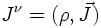CONSEQUENCES
1) The electromagnetic current is conserved: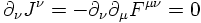2) The time derivative of the electric field in the fourth Maxwell equation guaranteeing local charge conservation leads also to the prediction of electromagnetic waves: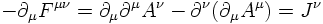In the absence of external electromagnetic currents and using the Lorentz gauge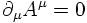one obtains for each compoenent of the four-potential (identified with the photon field) a Klein-Gordon equation for a massless particle: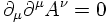2. Phase invariance of the Dirac field
3. The Aharanov-Bohm effect